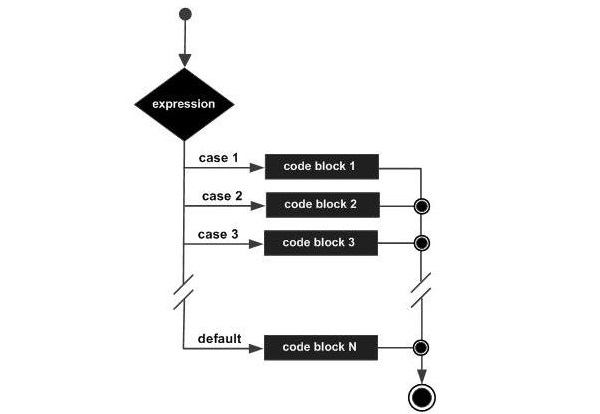# Clojure - Case Statement

Clojure offers the ‘case’ statement which is similar to the ‘switch’ statement available in the Java programming language. Following is the general form of the case statement.

## Syntax

```case expression
value1 statement #1
value2 statement #2
valueN statement #N
statement #Default
```

The general working of this statement is as follows −

• The expression to be evaluated is placed in the ‘case’ statement. This generally will evaluate to a value, which is used in the subsequent statements.

• Each value is evaluated against that which is passed by the ‘case’ expression. Depending on which value holds true, subsequent statement will be executed.

• There is also a default statement which gets executed if none of the prior values evaluate to be true.

Following diagram shows the flow of the ‘if’ statement.## Example

Following is an example of the ‘case’ statement in Clojure.

```(ns clojure.examples.hello
(:gen-class))

;; This program displays Hello World
(defn Example []
(def x 5)
(case x 5 (println "x is 5")
10 (println "x is 10")
(println "x is neither 5 nor 10")))
(Example)
```

In the above example, we are first initializing a variable ‘x’ to a value of 5. We then have a ‘case’ statement which evaluates the value of the variable ‘x’. Based on the value of the variable, it will execute the relevant case set of statements. The last statement is the default statement, if none of the previous statements are executed.

## Output

The above code produces the following output.

```x is 5
```
clojure_decision_making.htm